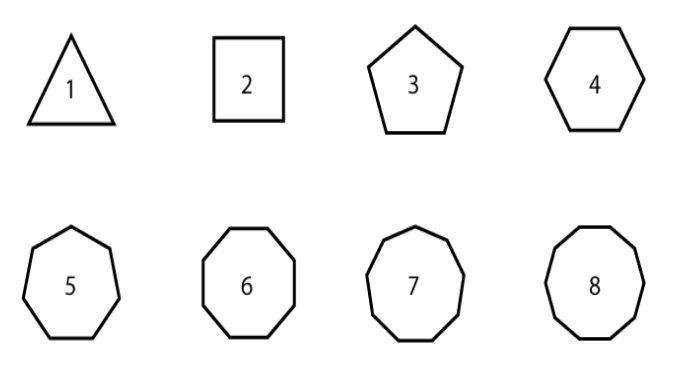# GRE Quantitative: Geometry – Polygons

Polygons always give a shiver to most students but you don’t need to be afraid of it. Polygon is no scary topic, in fact it is a really interesting and fun topic. Therefore this topic from the GRE Quantitative Reasoning section can be easily mastered by understanding the fundamental points and practicing problems.

Let us understand What polygons are and What its concepts are?

Any two-dimensional figure made up of three or more straight sides are known as polygons. The naming of the polygons is done according to the number of sides they are made of.Name of Polygon Number of sides
Triangle 3
Pentagon 5
Hexagon 6
Heptagon 7
Octagon 8
Nonagon 9
Decagon 10
Do-decagon 12
n-gon n

Properties of Polygons

• The sum of all the interior angles of a polygon having n- number of sides (n – 2)×180°. For example, the summation of the interior angles of a do-decagon will be :

(12 – 2) × 180°= 10 × (180°) = 1800°.

• Any polygon that has equal interior angles and equal sides is known as a regular polygon.
• The perimeter of a polygon = Summation of all the sides of the polygon
• The total sum of the exterior angles of any polygon = 360°.
• The area of a polygon = The region bounded by the polygon. Each polygon figure has different formulas specified for calculating their area.

Characteristics of Polygons

1. Triangles: This is the simplest form of polygon having only three sides. Properties of triangle:
• The summation of the two sides of a triangle is always greater than the third side. Similarly, the difference of two sides is always less than the third side.
• The greater is the angle of a triangle, the greater will be its corresponding side.
• The sum of the internal angles of a triangle is always supplementary.
• There are many types of triangles, namely; equilateral, isosceles, scalene, acute angle triangle, obtuse angled triangle, right angled triangle.
• The sum of angles in the triangle is 180 degrees.
• There are mainly six types of quadrilaterals, namely; square, rectangle, kite, parallelogram, trapezium and rhombus.
• A parallelogram is a type of quadrilateral where the opposite sides are parallel and congruent. The diagonals of a parallelogram bisects each other.The area of a parallelogram is the product of base and height.
• A parallelogram with only one set of parallel sides is known as a trapezium. The area of a trapezium is the average length of the parallel sides multiplied by height.
• Sum of internal angles as well as external angles of a quadrilateral is always equal to 360o.
1. Pentagon: Five straight sides together make up a pentagon
• The summation of the internal side of a pentagon is equal to 540o
• The angle made at the center of a regular pentagon formed by each side = 360o÷5 = 72o.
• Every individual interior angle of a regular pentagon = 108o
• There are two types of polygons, namely; regular and irregular. Curved shapes are not polygons.
• A regular polygon has equal length sides and equal angles between each side.
• An irregular polygon has unequal length sides and unequal angles between sides.

The polygons usually tested in the GRE exam are triangles and quadrilaterals. The quadrilaterals that are highly liked by GRE question makers are: Parallelograms, Squares, Rectangles and Kites.

BYJU’S will be glad to help you in your GRE preparation journey. You can ask for any assistance related to GRE from us by just giving a missed call at +918884544444, or you can drop an SMS. You can write to us at gre@byjus.com.